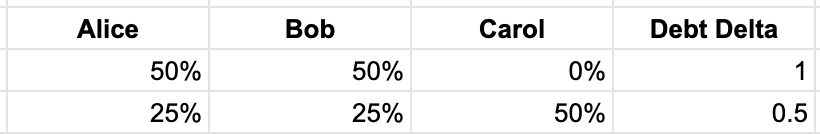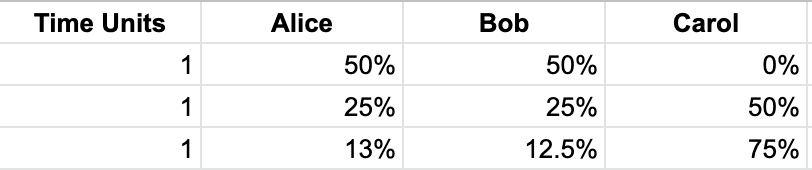# Time based staking rewards #58

Open
opened this issue Dec 3, 2019 · 4 comments
Open

# Time based staking rewards#58

opened this issue Dec 3, 2019 · 4 comments

###kaiynne commented Dec 3, 2019 • edited

 The current rewards calculation is snapshot based. With a single snapshot taken each week at ~08:00 UTC on Wednesday. The percentage of the global debt represented by each wallet is recorded and rewards are allocated proportionately. This mechanism presents an attack vector on the system as a free rider could potentially mint just before each snapshot, then burn immediately after, receiving rewards without risk. In practice this has not been a common strategy, though it has been observed. The main reasons for this are that most wallets do not retain sufficient Synths to fully clear their debt before each snapshot making this strategy less effective. In addition there are incentives to keeping sUSD or sETH in the depot and/or Uniswap, due to potential liquidity in the case of the depot or LP rewards for the sETH pool. However, if there is one pattern we have observed this year it is that any attack vector in the system will eventually be exploited, so rather than accept this latent risk and assume the current friction will not be automated away we have spent time on R&D to close the attack preemptively. This issue will outline a SIP that will change the mechanism for calculating rewards to rely on not only debt at the time of the snapshot but the average debt over the course of the period for each wallet. To understand how this mechanism works I will first provide an overview of the current debt register. To calculate rewards on-chain we must compress the information about the debt distribution to a few operations. With thousands of wallets minting and burning each week, storing the state of each wallet and calculating rewards would not be viable on-chain. Instead we use the fact that a mint or burn event has an equal impact on every other wallet in the system. To illustrate this, we will consider a three player game.At T0 Alice and Bob have 50% of the debt. At T1 Carol enters and doubles the global debt, reducing the debt of both Alice and Bob by 50%. The key is that the starting debt is irrelevant for either Alice or Bob, they will both be equally impacted by this change or debt delta. It also holds that no matter how many players are in the game any increase or decrease in the global debt by any player will impact each other player equally. Once we identify this property of the system we can then compress all mint and burn events debt deltas by tracking the cumulative debt delta, CDD which is the product of each debt delta. For any wallet we can determine their current debt by storing three values, the initial debt percentage of the global debt they represented, the CDD index number they entered the system at and the CDD index number now. Take the simple case of Alice: At T0 Alice enters the system as the only player with 100% of the debt. Between T1 and T4 Bob doubles the debt three times. T1: 100 | 0 T2: 50 | 50 T3: 25 | 75 T4: 12.5 | 87.5 Alice was 100% of the debt, and the CDD at T3 is 0.5 * 0.5 * 0.5 = .125 so we divide the CDD at T3 by the CDD at T0 multiplied by the initial debt percentage and we get .125/1 * 100% and we get 12.5% which is what the table above shows. Again, this holds for every case provided we have these three values. This now allows us to only store the CDD and the opening debt percentage for each wallet and from this information alone we can determine the debt of every wallet at any given time, specifically at the close of the period for the purpose of calculating rewards. This calculation also allows us to provide each wallet with an exact amount of debt that must be burned to exit the system at any time without tracking every event for every wallet individually. When it comes to calculating rewards we take the snapshot for all wallets at the close of the period as if they had requested to exit the system and we then calculate the percentage of the debt each wallet represents and its proportion of the rewards for that period. As mentioned above this is suboptimal as it means that a wallet that has minted since the start of the period will get the same rewards as one that mints in the block immediately before the snapshot. Increasing the number snapshots does not resolve this, as even with a high number of snapshots a system could be constructed to automate the minting and burning around each snapshot with the only cost being gas and some infrastructure. To close this attack vector we need to track the debt of each wallet over time throughout the period. But as mentioned above we need to be able to compress this information into a few operations to make the calculation viable on-chain. We need to record the time interval between each mint and burn in order to capture the impact of the changes to the debt register over time. This is a change to the current system where we discard the previous debt register information if a user mints of burns multiple times within a period, because currently we only care about the last mint/burn event. The calculations can be found here: https://docs.google.com/spreadsheets/d/12FLqU_q4vn57qm9ZwDyBUjrWZOBDagX00YRTOV5n-DM/edit#gid=0The table above shows a simple case of three events, in the case of Alice we can determine her average debt position over time with the following formula: Davg = (Initial Debt % * # of blocks elapsed * T0 Cumulative Debt Delta) + (Initial Debt % * # of blocks elapsed * T1 Cumulative Debt Delta) + (Initial Debt % * # of blocks elapsed * T2 Cumulative Debt Delta) Which can be factored to: Initial Debt % * SUM (# of blocks elapsed * CDD) Which allows us to create a new value that we increment with each mint/burn event to capture the impact that mint/burn had on all other wallets and for how long, allowing us to determine their average debt percentage over the period. We already store the debt register entry where the wallet last minted/burned and the debt percentage at that time. So now by combining this information with the time based CDD value from when they entered and the close of the period we can track all wallets. The issue is this calculation requires that the entry debt percentage remains static during the period, so if someone mints or burns their starting debt percentage will change. In order to resolve this we need to have a new variable where we can capture the accrued fee pool percentage for a wallet when it mints or burns and then we can begin to track the fee accruals from that point forward. This also handles the case where a wallet joins for the first time or exits the system completely. Because in both cases we simply write the accrued fees to this variable and then wait for their next mint/burn or the close of the fee period whichever comes first. So at the end of the fee period when a wallet claims fees we first read the accrued fee percentage variable, if this is zero then we calculate the fee percentage by looking at the entry debt percentage and multiplying that by the time based cumulative debt delta to work out the average percentage of the debt pool for that wallet during the fee period. We now have a system that more fairly distributes rewards but that allows a wallet to exit or enter the debt pool during the fee period and only lose the fees during the time that they have no debt. Once this proposal has been discussed and validated by the community I will write a SIP and the engineering team will determine the specific implementation within the system.

###ACE0999 commented Jan 17, 2020 • edited

Hello,

Following the brief discussion on discord, I layout here my argument as to why this method is not properly allocating the rewards to different participants. I first define in the below section the different components of the new method in order to pinpoint the main underlying weakness.

### Proposed Method System of Equations:

Debt Delta:
The proposed method relies on the DD (Debt Delta), which is nothing more then the total debt level (denoted D) in the previous period divided by the total debt of the current period. Note that for the first period (i.e. when i = 1) DD is set to 1.Cumulative Debt Delta:
The Cumulative Debt Delta (CDD) is iteratively calculated by multiplying the DD in the current period, by the CDD in the previous period. Note that for the first period (i.e. when i = 1 ) CDD is set to 1 as well:Now the CDD itself can be simplified further into the debt components as follows:Average Debt:
With the above definitions, we can show how the calculation proposed in this method, is able to calculate the sum of all weights for all the periods:
First we defineas being the debt of player 1 in time zero.So from the equation above, we can clearly see that if we have a constant debt for player X (i.e. player X does not gain or lose debt across the periods), the equation will work to compute the sum of all the weights for the periods spanning from 0 to T.

### Method Weakness:

My concern is that the debt itself for player X changes with time, because as other players gain/lose in the market, the debt of X will change dynamically. The above equation fails to capture this exact movement.
Example:
In the attached excel, I show how this miscalculation will take place in a simple example.

• Period 1: Alice and Bob mint 50$each, Carol 100$. Bob invests in a Synth, Alice and Carol stays in sUSD.

###ACE0999 commented Feb 16, 2020 • edited

 Hi @gloc90 , Thank you for taking the time to review this, I'm glad you understood the math I was trying and failing to explain, I tried several times on Discord but did not manage. I have left since few weeks back, so I'm not really up-to-date but last I remember front-running and snapshotting were systemic attack vectors , while this is more of a problem related to the proportional allocation of rewards amongst minters. I am not sure of the exploit though, if it exists or not, I didn't really think that far ahead on this, but I won't be surprised if it turns out to be a way to tilt rewards to one's advantage, as the numbers I used were simplistic, and the deviation could potentially be tilted to a much larger extent than the 2%. Thanks a lot for confirming my thoughts on this, I am not sure it is worth the effort though to think more on this, unless one day, we find someone is exploiting this loophole somehow.